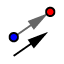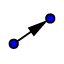# Translating by Vector <a,b> APS

## DIRECTIONS:

In the applet below, 1) Construct a triangle. A (2,1) B (4,1) C(3,4) 2) Use the VECTOR TOOLto construct any vector whose terminal points DOES NOT lie on top of its initial point. 3) Display the label of the triangle's 3 vertices and vector you've just constructed. Use the "Name and Value" option when showing the label. 4) Use the TRANSLATE BY VECTOR TOOLto translate the triangle by <-6,-4>

## 5)

Suppose the coordinates of a point P = (x, y). What is the image of P under a translation by vector with components <a, b>?

When you're done (or if you're unsure of something), feel free to check by watching the quick silent screencast below the applet.

## Quick (Silent) Demo

In the applet below, 1) Construct a quadrilateral A (-4, 1) B(-1,1) C (-1,5) D (-4, 5) 2) Use the VECTOR TOOLto construct any vector whose terminal points DOES NOT lie on top of its initial point. 3) Display the label of the triangle's 3 vertices and vector you've just constructed. Use the "Name and Value" option when showing the label. 4) Use the TRANSLATE BY VECTOR TOOLto translate the triangle by <0,-3> 5) Use the TRANSLATE BY VECTOR TOOLto translate the triangle by <5,0> 6)Use the TRANSLATE BY VECTOR TOOLto translate the triangle by <4,-5>

Translating the Quadrilateral ABCD by <0,-3> moved the pre-image

Select all that apply
• A
• B
• C
• D

Translating the Quadrilateral ABCD by <5,0> moved the pre-image

Select all that apply
• A
• B
• C
• D

Translating the Quadrilateral ABCD by <4,-5> moved the pre-image

Select all that apply
• A
• B
• C
• D